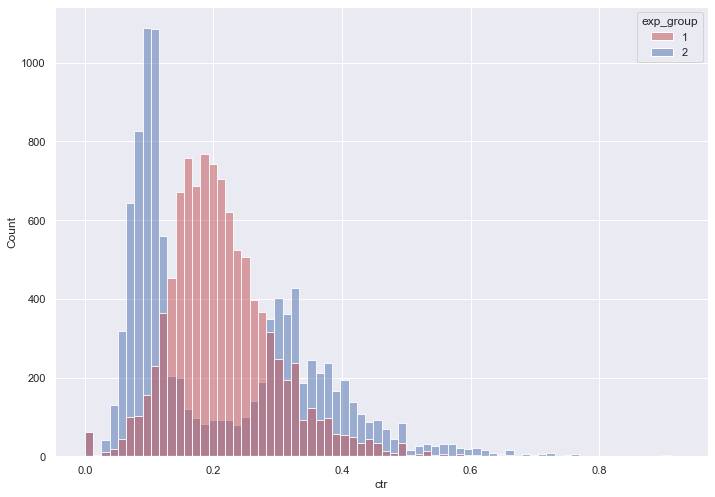This article is a continuation of the last article on A/B testing and a series of articles on my work on analytics.

Now let's analyze the results of the experiment that took place from 2022–05–24 to 2022–05–30 inclusive. Groups 2 and 1 were used for the experiment.

In group 2, one of the new algorithms for recommending posts was used, group 1 was used as a control.

The main hypothesis is that the new algorithm in the 2nd group will lead to an increase in CTR.

At the first step, as usual, we will download the necessary libraries.

```import pandas as pd
import pandahouse as ph
import numpy as np
import matplotlib.pyplot as plt
import seaborn as sns
from scipy import stats
```

Connecting to a data base in which a division into five groups has already been created earlier

```connection = {
'host': 'https://clickhouse.lab.karpov.courses',
'user': 'student',
'database': 'simulator_20220620'
}
```

We get only groups 2 and 3 from the database

```q = """
SELECT exp_group,
user_id,
sum(action = 'like') as likes,
sum(action = 'view') as views,
likes/views as ctr
FROM {db}.feed_actions
WHERE toDate(time) between '2022-05-24' and '2022-05-30'
and exp_group in (1,2)
GROUP BY exp_group, user_id
"""
```
```df = ph.read_clickhouse(q, connection=connection)
```
```df.groupby('exp_group').count()
```
user_id likes views ctr
exp_group
1 10079 10079 10079 10079
2 9952 9952 9952 9952
```sns.set(rc={'figure.figsize':(11.7,8.27)})

groups = sns.histplot(data = df,
x='ctr',
hue='exp_group',
palette = ['r', 'b'],
alpha=0.5,
kde=False)
```Next, you need to choose the analysis method and compare the SEC in two groups.

The distribution in the second group is not normal, so the use of a t-test is not recommended. But you can see how it will work:

```stats.ttest_ind(df[df.exp_group == 1].ctr,
df[df.exp_group == 2].ctr,
equal_var=False)
```
`Ttest_indResult(statistic=0.7094392041270485, pvalue=0.4780623130874935)`

The student's T-test showed that there are no differences. But I remind you once again that its use in this case is not recommended.

Now let's compare it with the Mann-Whitney test:

```stats.mannwhitneyu(df[df.exp_group == 1].ctr,
df[df.exp_group == 2].ctr,
alternative = 'two-sided')
```
`MannwhitneyuResult(statistic=56601260.5, pvalue=6.0376484617779035e-56)`

The Mann-Whitney criterion shows that the values do differ.

And at the end, we will use a Poisson bootstrap to calculate the difference in CTR.

```def bootstrap(likes1, views1, likes2, views2, n_bootstrap=2000):

poisson_bootstraps1 = stats.poisson(1).rvs(
(n_bootstrap, len(likes1))).astype(np.int64)

poisson_bootstraps2 = stats.poisson(1).rvs(
(n_bootstrap, len(likes2))).astype(np.int64)

globalCTR1 = (poisson_bootstraps1*likes1).sum(axis=1)/(poisson_bootstraps1*views1).sum(axis=1)

globalCTR2 = (poisson_bootstraps2*likes2).sum(axis=1)/(poisson_bootstraps2*views2).sum(axis=1)

return globalCTR1, globalCTR2
```
```likes1 = df[df.exp_group == 1].likes.to_numpy()
views1 = df[df.exp_group == 1].views.to_numpy()
likes2 = df[df.exp_group == 2].likes.to_numpy()
views2 = df[df.exp_group == 2].views.to_numpy()
```
```ctr1, ctr2 = bootstrap(likes1, views1, likes2, views2)

sns.histplot(ctr1)
sns.histplot(ctr2)
```
`<AxesSubplot:ylabel='Count'>`The difference between global CTR.

```sns.histplot(ctr2 - ctr1)
```
`<AxesSubplot:ylabel='Count'>`The T-test showed that there is no difference. But its use is not recommended, since the second distribution does not visually relate to the normal one. The use of Mann-Whitney and Poisson bootstrap methods showed that a significant difference was obtained. At the same time, it is important to note that the resulting sample, where a new algorithm for recommending posts was used, showed a decrease in CTR. In this connection, it is better not to use the new recommendation system.

That's it. In the next post, we will consider a different approach to conducting AB testing.